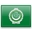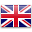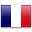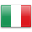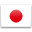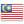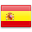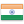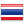VCE Further Mathematics - Gladstone Park

# VCE Further Mathematics

https://www.vcaa.vic.edu.au/curriculum/vce/vce-study-designs/furthermathematics/Pages/Index.aspx

Is this subject available for acceleration? YES

Further Mathematics has a strong emphasis on calculation, interpretation and analysis.

This link shows a selection of jobs that have some relation to the subject of: Further Mathematics

##### Units 1 and 2
• It covers the following topics: Data Analysis, Financial Mathematics, Matrices, Recursion, Networks, Algebra, Measurement and Linear Graphs
• Can be taken alone or in conjunction with Year 11 Mathematical Methods Units 1 and 2
• Designed for students who are intending to continue with VCE Further Mathematics Units 3 and 4

##### Units 3 and 4
• The Core (Unit 3) comprises of Data Analysis sand Recursion and Financial Modelling
• Unit 4 comprises of a selection of 2 modules from either Matrices, Networks, Geometry and Measurement and Graphs and Relations

A CAS Calculator is required for this subject

##### Skills
• construct and interpret graphical displays of data
• use a scatterplot to describe an observed association between two numerical variables
• solve related problems involving interest, appreciation and depreciation, loans, annuities and perpetuities
• gain an understanding of the different types of matrices, matrix operations and transition matrices
• define and represent undirected and directed networks

##### Associated subjects
• Biology
• Economics
• Accounting
• Psychology